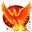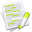#wx.lib.floatcanvas.FCObjects.Arc¶

Draws an arc of a circle, centered on point CenterXY, from the first point StartXY to the second EndXY.

The arc is drawn in an anticlockwise direction from the start point to the end point.

##Class Hierarchy¶Inheritance diagram for class Arc:

##Methods Summary¶

 __init__ Default class constructor. CalcBoundingBox Calculate the bounding box. Move Move the object by delta

##Class API¶

class Arc(XYObjectMixin, LineAndFillMixin, DrawObject)

Draws an arc of a circle, centered on point CenterXY, from the first point StartXY to the second EndXY.

The arc is drawn in an anticlockwise direction from the start point to the end point.

### Methods¶

__init__(self, StartXY, EndXY, CenterXY, LineColor = "Black", LineStyle = "Solid", LineWidth = 1, FillColor = None, FillStyle = "Solid", InForeground = False)

Default class constructor.

Parameters
• StartXY – start point, takes a 2-tuple, or a (2,) NumPy array of point coordinates

• EndXY

end point, takes a 2-tuple, or a (2,) NumPy array of point coordinates

• CenterXY

center point, takes a 2-tuple, or a (2,) NumPy array of point coordinates

• LineColor – see SetColor

• LineStyle – see SetLineStyle

• LineWidth – see SetLineWidth

• FillColor – see SetColor

• FillStyle – see SetFillStyle

• InForeground (boolean) – should object be in foreground

CalcBoundingBox(self)

Calculate the bounding box.

Move(self, Delta)

Move the object by delta

Parameters

Delta

delta is a (dx, dy) pair. Ideally a NumPy array of shape (2,)